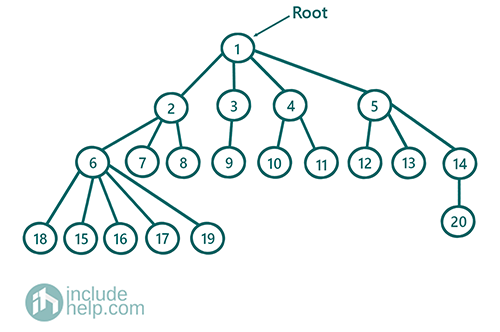# Generic Tree (N-array Tree)

In this article, we are going to see what the N-array tree is and how to represent the N-array tree efficiently? We have given here details on basic N-array tree understandings and as a follow-up, we have linked few problems on N-array tree. Also, we have discussed here how to traverse an N-array tree. The N-array tree is also popularly known as Generic Tree.
Submitted by Radib Kar, on July 30, 2020

## What is N-array Tree?

N-array tree is known as "generic tree" where a parent can have at most n number of children, where n is any non-negative integer. In other words, the N-array tree is an acyclic undirected graph. To extend the idea of this generic tree we can say that a binary tree is a 2-array tree where each parent can have a maximum of two children. Moreover, in an n-array tree, there is only one single root as like other kinds of trees.

Below is an example of an n-array tree:The above is an example of an n-array tree. Where the root has the value 1 and it has four children namely 2, 3, 4, 5 which in turns have a different number of children.

## How to represent an N-array tree?

In the binary tree, there was at most two children and that's why we could do like left and right child which may be NULL or not. But in an n-array tree, it's not possible to give each child a name to hardcode like left, right etc.

So, that means we need an array to store the children. Now, if we keep a static array of size n (n be the max no of children any tree node can have), then there can be cases that many nodes don't have even half of n no child nodes. So, then it will be wastage of space only.

```int max_child_no=n
class TreeNode{
int val;
TreeNode* children[max_child_no];
}
```

So, the above structure will be only wastage of memory, instead of that, we may only store as many children as needed. So better keep a dynamic array to store children. Let's use vector for that. So a better representation of N-array Tree would be like below:

```class TreeNode{
int val;
vector<TreeNode*> children;
}
```

## Traversing an N-array Tree

### 1) Depth First Traversal

This is quite similar to the DFS of the graph. Here we traverse the depth-first instead of breadth. The algorithm for depth-first traversal is:

```DFS(TreeNode* root){
if(root is NULL)
return
if(root is a leaf node)
print the root and return
for each child of the root:
DFS(child node)
End for
}
```
```#include <bits/stdc++.h>
using namespace std;

class TreeNode {
public:
int val;
vector<TreeNode*> children;
TreeNode(int v)
{
val = v;
}
TreeNode(int v, int n)
{
val = v;
children = vector<TreeNode*>(n, NULL);
}
};

void dfs(TreeNode* root)
{
if (!root)
return;

if (root->children.size() == 0) {
cout << root->val << " ";
return;
}

cout << root->val << " ";

for (int i = 0; i < root->children.size(); i++) {
dfs(root->children[i]);
}
}

int main()
{
TreeNode* root = new TreeNode(1, 4);

root->children = new TreeNode(2, 3);
root->children = new TreeNode(3, 1);
root->children = new TreeNode(4, 2);
root->children = new TreeNode(5, 3);

root->children->children = new TreeNode(6, 5);
root->children->children = new TreeNode(7);
root->children->children = new TreeNode(8);

root->children->children = new TreeNode(9);

root->children->children = new TreeNode(10);
root->children->children = new TreeNode(11);

root->children->children = new TreeNode(12);
root->children->children = new TreeNode(13);
root->children->children = new TreeNode(14, 1);

root->children->children->children = new TreeNode(15);
root->children->children->children = new TreeNode(16);
root->children->children->children = new TreeNode(17);
root->children->children->children = new TreeNode(18);
root->children->children->children = new TreeNode(19);

root->children->children->children = new TreeNode(20);

cout << "Depth First Traversal" << endl;
dfs(root);

return 0;
}
```

Output:

```Depth First Traversal
1 2 6 15 16 17 18 19 7 8 3 9 4 10 11 5 12 13 14 20
```

To traverse in level order we need to do Breadth-First Search like the graph. For that, we will use the queue as we did in level order traversal for a binary tree.

Algorithm:

```1)  Define a queue q
2)  EnQueue root to the queue
3)  While the queue is not empty:
Current Node=DeQueue
Print current Node
EnQueue all child into the queue q
End While
```
```#include <bits/stdc++.h>
using namespace std;

class TreeNode {
public:
int val;
vector<TreeNode*> children;
TreeNode(int v)
{
val = v;
}
TreeNode(int v, int n)
{
val = v;
children = vector<TreeNode*>(n, NULL);
}
};

void bfs(TreeNode* root)
{
if (!root)
return;

queue<TreeNode*> q;
q.push(root);
while (!q.empty()) {

TreeNode* temp = q.front();
q.pop();

cout << temp->val << " ";

for (int i = 0; i < temp->children.size(); i++) {
q.push(temp->children[i]);
}
}
}

int main()
{
TreeNode* root = new TreeNode(1, 4);

root->children = new TreeNode(2, 3);
root->children = new TreeNode(3, 1);
root->children = new TreeNode(4, 2);
root->children = new TreeNode(5, 3);

root->children->children = new TreeNode(6, 5);
root->children->children = new TreeNode(7);
root->children->children = new TreeNode(8);

root->children->children = new TreeNode(9);

root->children->children = new TreeNode(10);
root->children->children = new TreeNode(11);

root->children->children = new TreeNode(12);
root->children->children = new TreeNode(13);
root->children->children = new TreeNode(14, 1);

root->children->children->children = new TreeNode(15);
root->children->children->children = new TreeNode(16);
root->children->children->children = new TreeNode(17);
root->children->children->children = new TreeNode(18);
root->children->children->children = new TreeNode(19);

root->children->children->children = new TreeNode(20);

cout << "Level wise traversal\n";
bfs(root);

return 0;
}
```

Output:

```Level wise traversal
1 2 3 4 5 6 7 8 9 10 11 12 13 14 15 16 17 18 19 20
```